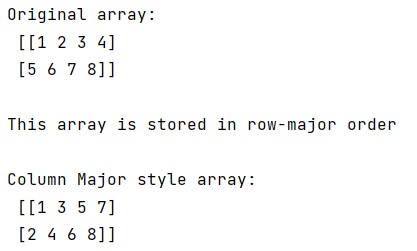# NumPy Array: Row major and column major

Learn about the NumPy row major and column major order of reading a NumPy order.
Submitted by Pranit Sharma, on March 05, 2023

NumPy is an abbreviated form of Numerical Python. It is used for different types of scientific operations in python. Numpy is a vast library in python which is used for almost every kind of scientific or mathematical operation. It is itself an array which is a collection of various methods and functions for processing the arrays.

## Row Major and Column Major in NumPy Array

In numpy, the data in an array is stored in row-major order. Row major order is nothing but a way of representing the elements of a multi-dimensional array in sequential memory. Here, elements are stored/arranged sequentially row by row which means it fills all the elements of the first row followed second till all the elements of the last row are stored.

However, column-major order is another order for representing the arrangement of a numpy multi-dimensional array but if we change the order from row-major to column-major, it does not change the way of storing the elements. It will read out the elements in column-major order.

In column-major order, the elements are read from a sequential memory column-by-column. This means that all the values of the first column are followed by the second column till the last column is read.

Let us understand with the help of an example,

## Python code for NumPy row major and column major order of reading a NumPy order

```# Import numpy
import numpy as np

# Creating a numpy array
arr = np.array([[1,2,3,4], [5,6,7,8]])

# Display Original array
print("Original array:\n",arr,"\n")

print("This array is stored in row-major order\n")

# Changing shape and order of array
arr = np.array([1, 2, 3, 4, 5, 6, 7, 8])
arr = arr.reshape(2,4,order = 'F')

# Display result
print("Column Major style array:\n",arr,"\n")
```

Output:Languages: » C » C++ » C++ STL » Java » Data Structure » C#.Net » Android » Kotlin » SQL
Web Technologies: » PHP » Python » JavaScript » CSS » Ajax » Node.js » Web programming/HTML
Solved programs: » C » C++ » DS » Java » C#
Aptitude que. & ans.: » C » C++ » Java » DBMS
Interview que. & ans.: » C » Embedded C » Java » SEO » HR
CS Subjects: » CS Basics » O.S. » Networks » DBMS » Embedded Systems » Cloud Computing
» Machine learning » CS Organizations » Linux » DOS
More: » Articles » Puzzles » News/Updates